Documentation

## Patch Antenna Array for FMCW Radar

This example shows how to model a 77 GHz 2x4 antenna array for Frequency-Modulated Continuous-Wave (FMCW) radar applications. The presence of antennas and antenna arrays in and around vehicles has become a commonplace with the introduction of wireless collision detection, collision avoidance, and lane departure warning systems. The two frequency bands considered for such systems are centered around 24 GHz and 77 GHz, respectively. In this example, we will investigate the microstrip patch antenna as a phased array radiator. The dielectric substrate is air.

This example requires the Antenna Toolbox™.

### Antenna Array Design

The FMCW antenna array is intended for a forward radar system designed to look for and prevent a collision. Therefore, A cosine antenna pattern is an appropriate choice for the initial design since it does not radiate any energy backwards. Assume that the radar system operates at 77 GHz with a 700 MHz bandwidth.

```fc = 77e9; fmin = 73e9; fmax = 80e9; vp = physconst('lightspeed'); lambda = vp/fc; cosineantenna = phased.CosineAntennaElement; cosineantenna.FrequencyRange = [fmin fmax]; pattern(cosineantenna,fc) ```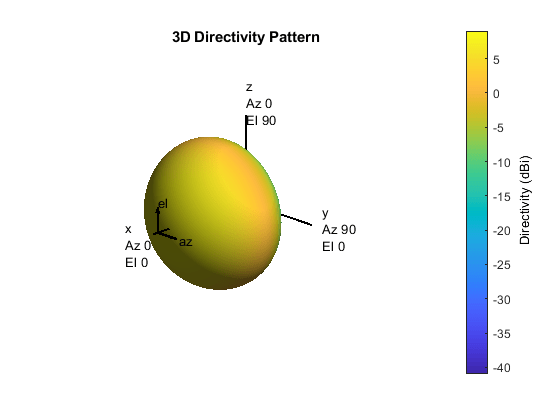The array itself needs to be mounted on or around the front bumper. The array configuration we investigate is a 2 X 4 rectangular array, similar to what is mentioned in . Such a design has bigger aperture along azimuth direction thus providing better azimuth resolution.

```Nrow = 2; Ncol = 4; fmcwCosineArray = phased.URA; fmcwCosineArray.Element = cosineantenna; fmcwCosineArray.Size = [Nrow Ncol]; fmcwCosineArray.ElementSpacing = [0.5*lambda 0.5*lambda]; pattern(fmcwCosineArray,fc) ```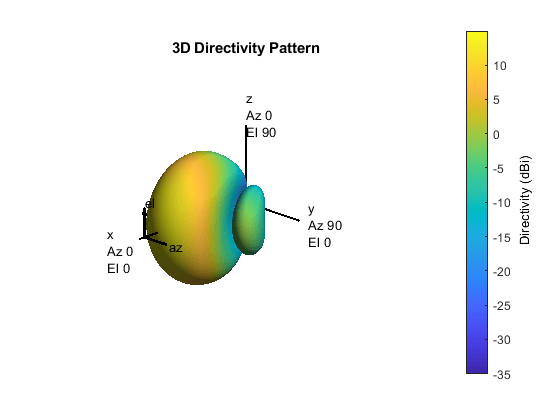### Design Realistic Patch Antenna

The Antenna Toolbox has several antenna elements that could provide hemispherical coverage and resemble a pattern of cosine shape. Choose a patch antenna element with typical radiator dimensions. The patch length is approximately half-wavelength at 77 GHz and the width is 1.5 times the length to improving the bandwidth. The ground plane is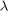on each side and the feed offset from center in the direction of the patch length is about a quarter of the length.

```patchElement = patchMicrostrip; patchElement.Length = 0.49*lambda; patchElement.Width = 1.5*patchElement.Length; patchElement.GroundPlaneLength = lambda; patchElement.GroundPlaneWidth = lambda; patchElement.Height = 0.01*lambda; patchElement.FeedOffset = [patchElement.Length/4 0]; ```

Because the default patch antenna geometry has its maximum radiation directed towards zenith, rotate the patch antenna by 90 degrees about the y-axis so that the maximum would now occur along the x-axis.

```patchElement.Tilt = 90; patchElement.TiltAxis = [0 1 0]; ```

### Isolated Patch Antenna 3D Pattern and Resonance

Plot the pattern of the patch antenna at 77 GHz. The patch is a medium gain antenna with the peak directivity around 6-9 dBi.

```myFigure = gcf; myFigure.Color = 'w'; pattern(patchElement,fc) ```The patch is radiating in the correct mode with a pattern maximum at 0 degrees azimuth and 0 degrees elevation. Since the initial dimensions are approximations, it is important to verify the antenna's input impedance behavior.

```Numfreqs = 21; freqsweep = unique([linspace(fmin,fmax,Numfreqs) fc]); impedance(patchElement,freqsweep); ```According to the figure, the patch antenna has its first resonance (parallel resonance) at 74 GHz. It is a common practice to shift this resonance to 77 GHz by scaling the length of the patch.

```act_resonance = 74e9; lambda_act = vp/act_resonance; scale = lambda/lambda_act; patchElement.Length = scale*patchElement.Length; ```

Next is to check the reflection coefficient of the patch antenna to confirm a good impedance match. It is typical to consider the value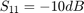as a threshold value for determining the antenna bandwidth.

```s = sparameters(patchElement,freqsweep); rfplot(s,'m-.') hold on line(freqsweep,ones(1,numel(freqsweep))*-10,'LineWidth',1.5) hold off ```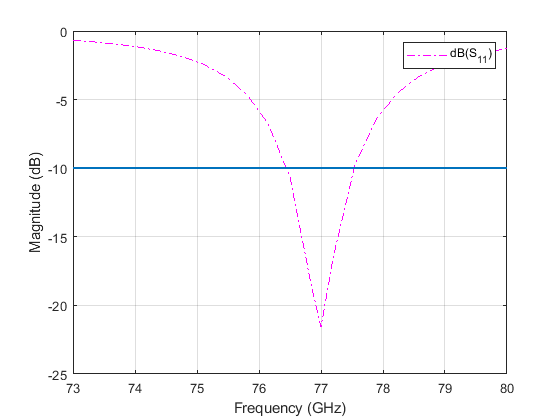The deep minimum at 77 GHz indicates a good match to 50. The antenna bandwidth is slightly greater than 1 GHz. Thus, the frequency band is from 76.5 GHz to 77.5 GHz.

Finally, check if the pattern at the edge frequencies of the band meets the design. This is a good indication whether the pattern behaves the same across the band. The patterns at 76.5 GHz and 77.6 GHz are shown below.

```pattern(patchElement,76.5e9) ```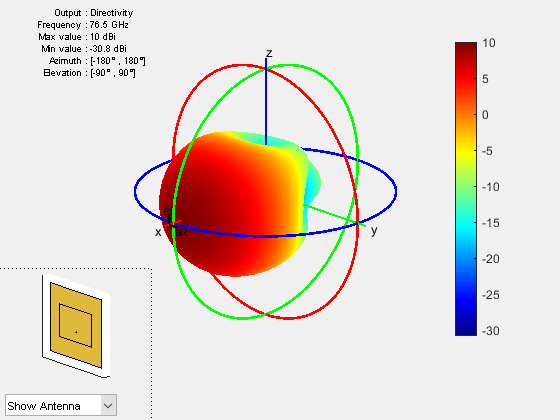```pattern(patchElement,77.6e9) ```In general, it is a good practice to check pattern behavior over the frequency band of interest.

### Create Array from Isolated Radiators and Plot Pattern

Next, creates a uniform rectangular array (URA) with the patch antenna. The spacing is chosen to be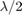, whereis the wavelength at the upper frequency of the band (77.6 GHz).

```fc2 = 77.6e9; lambda_fc2 = vp/77.6e9; fmcwPatchArray = phased.URA; fmcwPatchArray.Element = patchElement; fmcwPatchArray.Size = [Nrow Ncol]; fmcwPatchArray.ElementSpacing = [0.5*lambda_fc2 0.5*lambda_fc2]; ```

The following figure shows the pattern of the resulting patch antenna array. The pattern is computed using a 5 degree separation in both azimuth and elevation.

```az = -180:5:180; el = -90:5:90; %clf pattern(fmcwPatchArray,fc,az,el) ```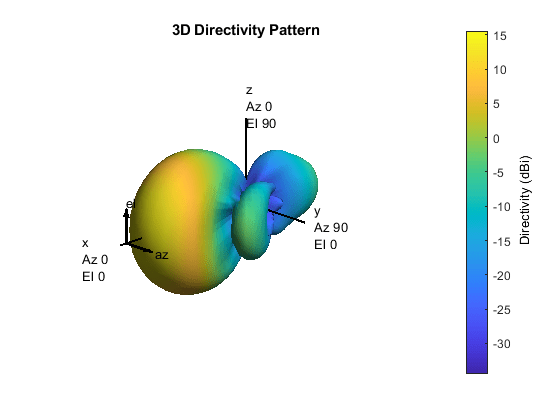Plots below compare the pattern variation in 2 orthogonal planes for the patch antenna array and the cosine element array. Note that both arrays ignore mutual coupling effect.

First, plot the patterns along the azimuth direction.

```patternAzimuth(fmcwPatchArray,fc) hold on patternAzimuth(fmcwCosineArray,fc) p = polarpattern('gco'); p.LegendLabels = {'Patch','Cosine'}; ```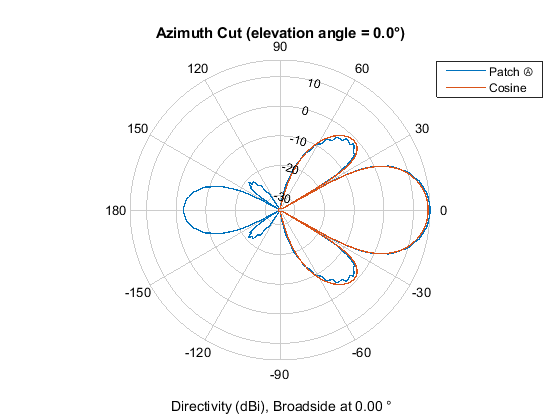Then, plot the patterns along the elevation direction.

```%clf patternElevation(fmcwPatchArray,fc) hold on patternElevation(fmcwCosineArray,fc) p = polarpattern('gco'); p.LegendLabels = {'Patch','Cosine'}; ```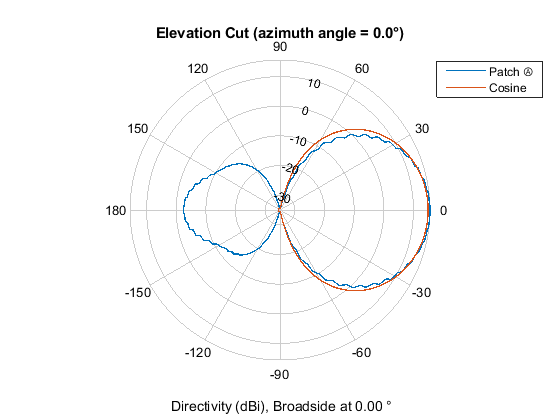The figures show that both arrays have similar pattern behavior around the main beam in the elevation plane (azimuth = 0 deg). The patch-element array has a significant backlobe as compared to the cosine-element array.

### Conclusions

This example starts the design of an antenna array for FMCW radar with an ideal cosine antenna and then uses a patch antenna to form the real array. The example compares the patterns from the two arrays to show the design tradeoff. From the comparison, it can be seen that using the isolated patch element is a useful first step in understanding the effect that a realistic antenna element would have on the array pattern.

However, analysis of realistic arrays must also consider mutual coupling effect. Since this is a small array (8 elements in 2x4 configuration), the individual element patterns in the array environment could be distorted significantly. As a result it is not possible to replace the isolated element pattern with an embedded element pattern, as shown in the Modeling Mutual Coupling in Large Arrays Using Embedded Element Pattern example. A full-wave analysis must be performed to understand the effect of mutual coupling on the overall array performance.

### Reference

 R. Kulke, et al. 24 GHz Radar Sensor Integrates Patch Antennas, EMPC 2005 `http://empire.de/main/Empire/pdf/publications/2005/26-doc-empc2005.pdf`